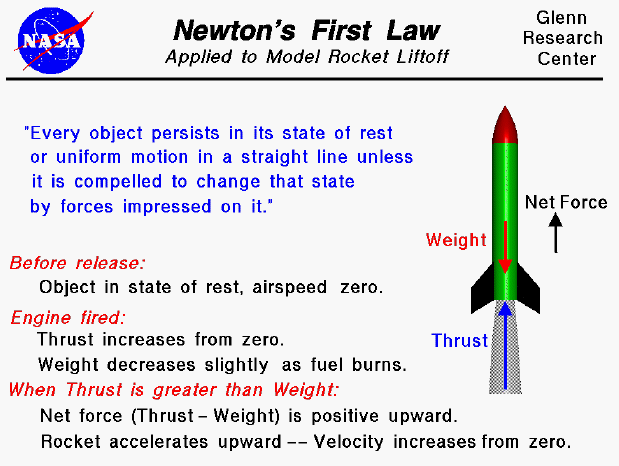Sir Isaac Newton first presented his three laws of motion in the "Principia Mathematica Philosophiae Naturalis" in 1686. His first law states that every object will remain at rest or in uniform motion in a straight line unless compelled to change its state by the action of an external force. This is normally taken as the definition of inertia. The key point here is that if there is no net force acting on an object (if all the external forces cancel each other out) then the object will maintain a constant velocity. If that velocity is zero, then the object remains at rest. And if an additional external force is applied, the velocity will change because of the force.

A model rocket lifting off from the launch pad is a good example of this principle. Just prior to engine ignition, the velocity of the rocket is zero and the rocket is at rest. If the rocket is sitting on its fins, the weight of the rocket is balanced by the re-action of the earth to the weight as described by Newton's third law of motion. There is no net force on the object, and the rocket would remain at rest indefinitely. When the engine is ignited, the thrust of the engine creates an additional force opposed to the weight. As long as the thrust is less than the weight, the combination of the thrust and the re-action force through the fins balance the weight and there is no net external force and the rocket stays on the pad. When the thrust is equal to the weight, there is no longer any re-action force through the fins, but the net force on the rocket is still zero. When the thrust is greater than the weight, there is a net external force equal to the thrust minus the weight, and the rocket begins to rise. The velocity of the rocket increases from zero to some positive value under the acceleration produced by the net external force. But as the rocket velocity increases, it encounters air resistance, or drag, which opposes the motion and increases as the square of the velocity. The thrust of the rocket must be greater than the weight plus the drag for the rocket to continue accelerating. If the thrust becomes equal to the weight plus the drag, the rocket will continue to climb at a fixed velocity, but it will not accelerate.Guided Tours

Back to top

Go to...

Beginner's Guide Home Page

byTom Benson
Please send suggestions/corrections to: benson@grc.nasa.gov